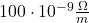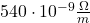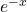## PhyMNS

Iron has Resistivity ofHSS has Resistivity of• Why is the transmission of a material in the proportion of, why not proportional, on what is the factor dependent atom size?
• Is glass on both sides coated? When yes why, one could also do one coating with more than one layer on one side?
• How can we calculate which material thickness absorbs which electromagnetic waves?
• why is silver more conductive than copper?
• Are there areas where iron is used in nanotechnologies? When yes where, when no why not?
• Why is impure silicon more conductive than pure silicon?
• How can one calculate the resistivity of a steel?
• How can one calculate how good one metal layer holds on the other one?